This is a fixed-text formatted version of a Jupyter notebook

# Source detection with Gammapy¶

## Context¶

The first task in a source catalogue production is to identify significant excesses in the data that can be associated to unknown sources and provide a preliminary parametrization in term of position, extent, and flux. In this notebook we will use Fermi-LAT data to illustrate how to detect candidate sources in counts images with known background.

Objective: build a list of significant excesses in a Fermi-LAT map

## Proposed approach¶

This notebook show how to do source detection with Gammapy using the methods available in gammapy.estimators. We will use images from a Fermi-LAT 3FHL high-energy Galactic center dataset to do this:

• perform adaptive smoothing on counts image

• produce 2-dimensional test-statistics (TS)

• run a peak finder to detect point-source candidates

• compute Li & Ma significance images

• estimate source candidates radius and excess counts

Note that what we do here is a quick-look analysis, the production of real source catalogs use more elaborate procedures.

We will work with the following functions and classes:

• gammapy.maps.WcsNDMap

• gammapy.estimators.ASmoothEstimator

• gammapy.estimators.TSMapEstimator

• ~gammapy.estimators.utils.find_peaks

## Setup¶

As always, let’s get started with some setup …

:

%matplotlib inline
import matplotlib.pyplot as plt
import matplotlib.cm as cm
from gammapy.maps import Map
from gammapy.estimators import (
ASmoothMapEstimator,
TSMapEstimator,
)
from gammapy.estimators.utils import find_peaks
from gammapy.datasets import MapDataset
from gammapy.catalog import SOURCE_CATALOGS
from gammapy.modeling.models import (
BackgroundModel,
SkyModel,
PowerLawSpectralModel,
PointSpatialModel,
)
from gammapy.irf import PSFMap, EnergyDependentTablePSF
from astropy.coordinates import SkyCoord
import astropy.units as u
import numpy as np


We first read in the counts cube and sum over the energy axis:

:

counts = Map.read(
"$GAMMAPY_DATA/fermi-3fhl-gc/fermi-3fhl-gc-counts-cube.fits.gz" ) background = Map.read( "$GAMMAPY_DATA/fermi-3fhl-gc/fermi-3fhl-gc-background-cube.fits.gz"
)
background = BackgroundModel(background, datasets_names=["fermi-3fhl-gc"])

"$GAMMAPY_DATA/fermi-3fhl-gc/fermi-3fhl-gc-exposure-cube.fits.gz" ) # unit is not properly stored on the file. We add it manually exposure.unit = "cm2s" mask_safe = counts.copy(data=np.ones_like(counts.data).astype("bool")) psf = EnergyDependentTablePSF.read( "$GAMMAPY_DATA/fermi-3fhl-gc/fermi-3fhl-gc-psf-cube.fits.gz"
)
psfmap = PSFMap.from_energy_dependent_table_psf(psf)

dataset = MapDataset(
counts=counts,
models=[background],
exposure=exposure,
psf=psfmap,
name="fermi-3fhl-gc",
)

dataset = dataset.to_image()

/Users/terrier/Code/gammapy-dev/gammapy-docs/build/v0.17/gammapy/gammapy/utils/interpolation.py:163: RuntimeWarning: overflow encountered in log
return np.log(values)


For visualisation purpose it can be nice to look at a smoothed counts image. This can be performed using the adaptive smoothing algorithm from Ebeling et al. (2006).

In the following example the threshold argument gives the minimum significance expected, values below are clipped.

:

%%time
scales = u.Quantity(np.arange(0.05, 1, 0.05), unit="deg")
smooth = ASmoothMapEstimator(threshold=3, scales=scales)
images = smooth.run(dataset)

plt.figure(figsize=(15, 5))

CPU times: user 1.26 s, sys: 551 ms, total: 1.81 s
Wall time: 1.82 s

:

(<Figure size 1080x360 with 2 Axes>,
<matplotlib.axes._subplots.WCSAxesSubplot at 0x11fa74710>,
<matplotlib.colorbar.Colorbar at 0x11d0395f8>)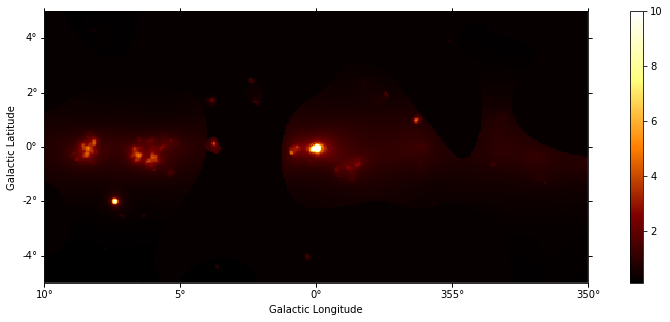## TS map estimation¶

The Test Statistic, TS = 2 ∆ log L (Mattox et al. 1996), compares the likelihood function L optimized with and without a given source. The TS map is computed by fitting by a single amplitude parameter on each pixel as described in Appendix A of Stewart (2009). The fit is simplified by finding roots of the derivative of the fit statistics (default settings use Brent’s method).

We first need to define the model that will be used to test for the existence of a source. Here, we use a point source.

:

spatial_model = PointSpatialModel()
spectral_model = PowerLawSpectralModel(index=2)
model = SkyModel(spatial_model=spatial_model, spectral_model=spectral_model)

:

%%time
estimator = TSMapEstimator(model)
images = estimator.run(dataset)

CPU times: user 9.7 s, sys: 419 ms, total: 10.1 s
Wall time: 10.1 s


### Plot resulting images¶

:

plt.figure(figsize=(15, 5))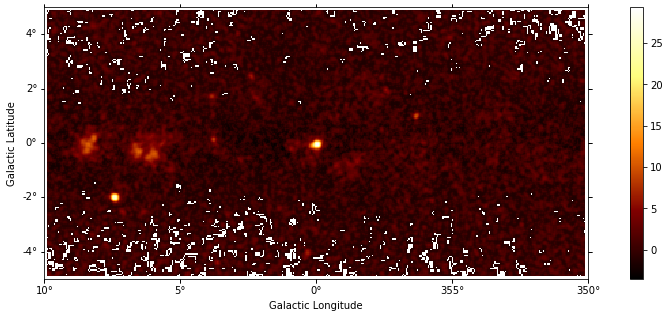:

plt.figure(figsize=(15, 5))

/Users/terrier/Code/anaconda3/envs/gammapy-dev/lib/python3.7/site-packages/matplotlib/colors.py:527: RuntimeWarning: invalid value encountered in less
xa[xa < 0] = -1
/Users/terrier/Code/anaconda3/envs/gammapy-dev/lib/python3.7/site-packages/matplotlib/colors.py:527: RuntimeWarning: invalid value encountered in less
xa[xa < 0] = -1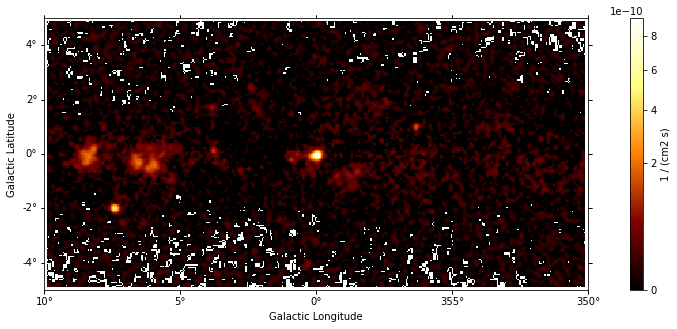:

plt.figure(figsize=(15, 5))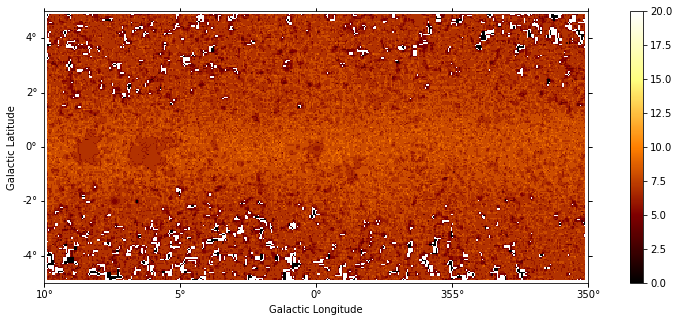## Source candidates¶

Let’s run a peak finder on the sqrt_ts image to get a list of point-sources candidates (positions and peak sqrt_ts values). The find_peaks function performs a local maximun search in a sliding window, the argument min_distance is the minimum pixel distance between peaks (smallest possible value and default is 1 pixel).

:

sources = find_peaks(images["sqrt_ts"], threshold=8, min_distance=1)
nsou = len(sources)
sources

:

Table length=9
degdeg
float64int64int64float64float64
29.36520199266.38470-29.01322
26.8175260272.43197-23.54282
11.056993270.40919-23.47797
10.8793299271.11366-21.72027
10.80136103270.82028-21.79615
10.3398092270.15899-23.98049
10.0943194271.37469-21.79916
9.7537273119263.18257-31.52587
8.4524124102268.46711-25.63326
:

# Plot sources on top of significance sky image
plt.figure(figsize=(15, 5))

ax.scatter(
sources["ra"],
sources["dec"],
transform=plt.gca().get_transform("icrs"),
color="none",
edgecolor="w",
marker="o",
s=600,
lw=1.5,
);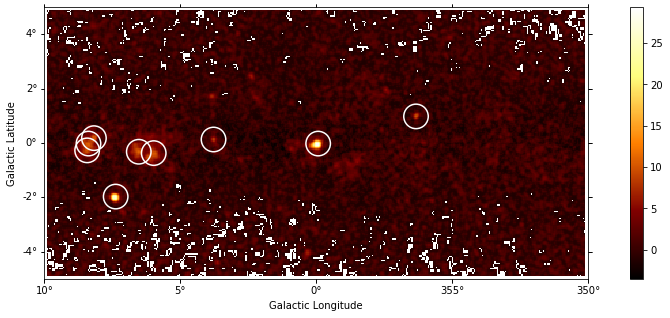Note that we used the instrument point-spread-function (PSF) as kernel, so the hypothesis we test is the presence of a point source. In order to test for extended sources we would have to use as kernel an extended template convolved by the PSF. Alternatively, we can compute the significance of an extended excess using the Li & Ma formalism, which is faster as no fitting is involve.

## What next?¶

In this notebook, we have seen how to work with images and compute TS and significance images from counts data, if a background estimate is already available.

Here’s some suggestions what to do next:

• Look how background estimation is performed for IACTs with and without the high-level interface in analysis_1 and analysis_2 notebooks, respectively

• Learn about 2D model fitting in the modeling 2D notebook

• find more about Fermi-LAT data analysis in the fermi_lat notebook

• Use source candidates to build a model and perform a 3D fitting (see analysis_3d, analysis_mwl notebooks for some hints)

[ ]: